# Dynamic Arrays

Dynamic array formulas, entered into a single cell, fill multiple cells. This behavior in Excel 365/2021 is called spilling.

### Formula Example

1. Fix the reference to cell E2 by placing a \$ symbol in front of the column letter and row number.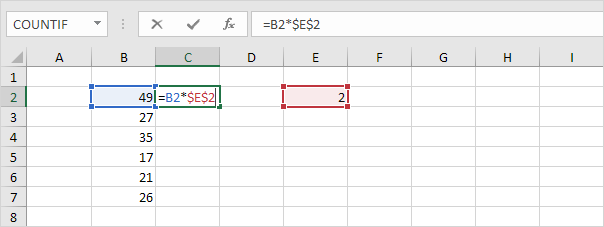2. To quickly copy the formula in cell C2 to the other cells, select cell C2, click on the lower right corner of cell C2 and drag it down to cell C7.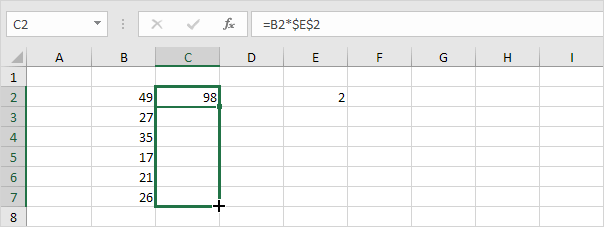Check:Explanation: the absolute reference (\$E\$2) stays the same, while the relative reference (B2) changes to B3, B4, B5, B6 and B7.

3. If you have Excel 365 or Excel 2021, simply enter the dynamic array formula shown below into cell C2.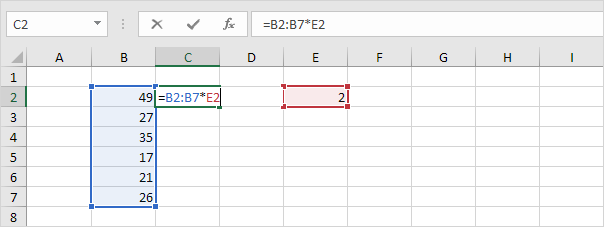4. Press Enter.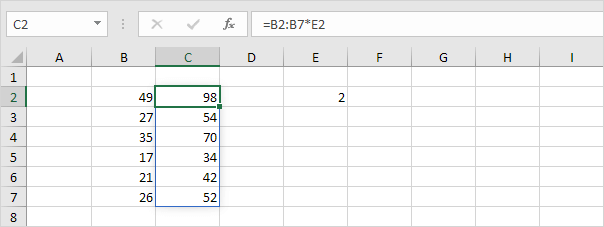Explanation: this dynamic array (DA) formula, entered into cell C2, fills multiple cells. This behavior in Excel 365/2021 is called spilling. If you select a cell in the range C2:C7, a blue border appears.

5. If you select a cell in the range C3:C7, the formula in the formula bar will be greyed out.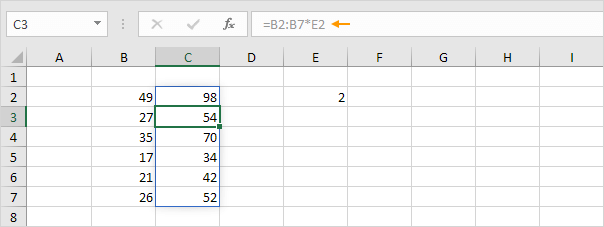### Dynamic Array Functions

1. The SORT function below sorts by the second column, in ascending order.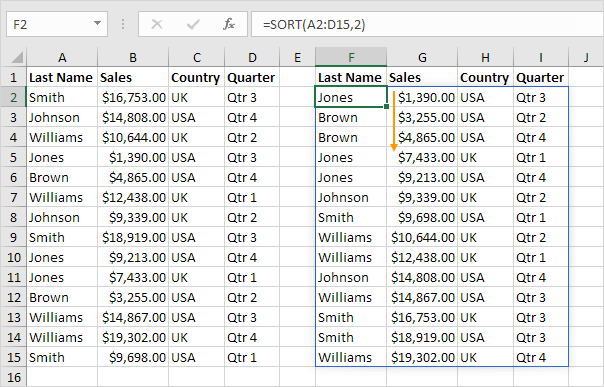Note: this dynamic array function, entered into cell F2, fills multiple cells. Wow!

2. The FILTER function below extracts all USA records.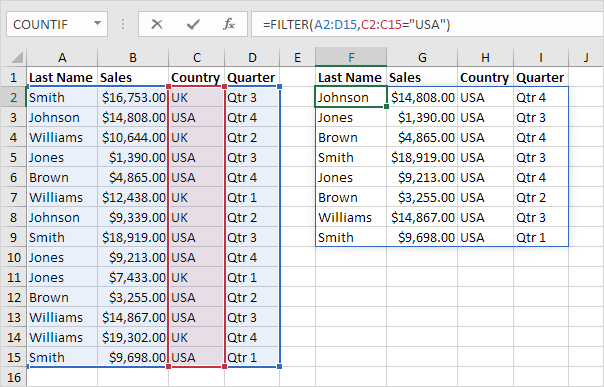3. The RANDARRAY function below generates random decimal numbers between 0 and 1. The array below consists of 5 rows and 2 columns.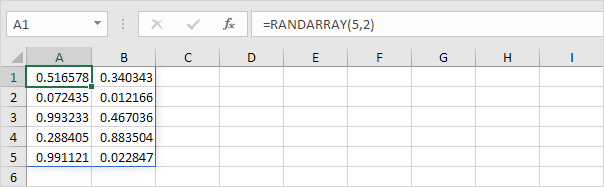4. The SEQUENCE function below generates a two-dimensional array. Rows = 7, Columns = 4, Start = 0, Step = 5.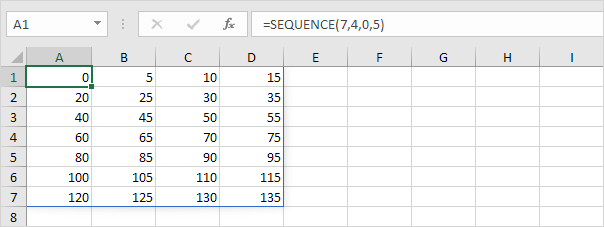5. The UNIQUE function below (with no extra arguments) extracts unique values.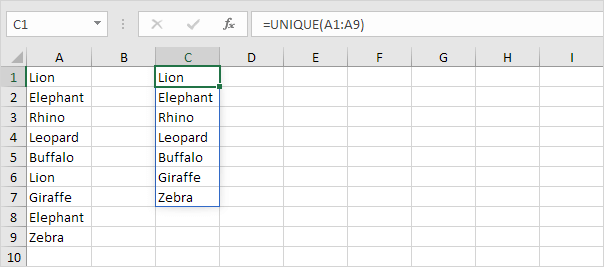6. The XLOOKUP function below looks up the ID and returns the first name, last name and salary.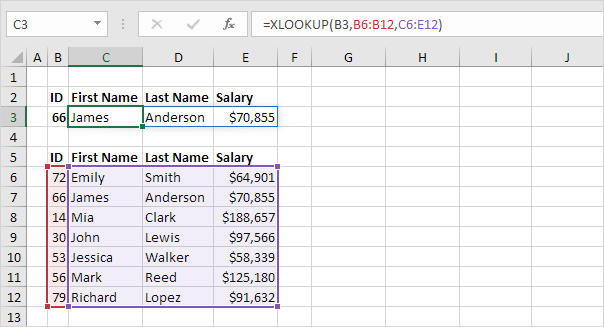Note: if you have Excel 365 or Excel 2021, use XLOOKUP instead of VLOOKUP. The XLOOKUP function is easier to use and has some additional advantages.

7. The SORTBY function sorts a range based on the values in a corresponding range.Note: use 1 to sort in ascending order, use -1 to sort in descending order.

### Old Array Formulas

Old array formulas still work. The good news is, if you have Excel 365 or Excel 2021, you don't have to press CTRL + SHIFT + ENTER anymore. Below you can find a few examples.

1. A traditional array formula finished by pressing CTRL + SHIFT + ENTER.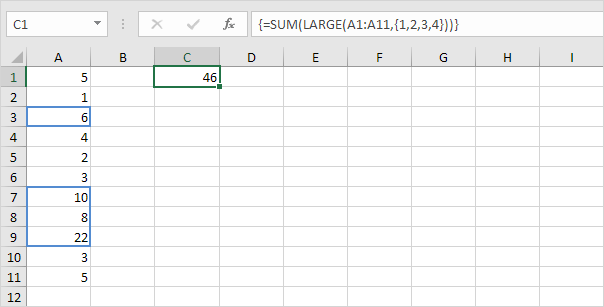Explanation: this formula sums the 4 largest numbers.

2. The same formula in Excel 365/2021 finished by simply pressing Enter. Bye bye curly braces.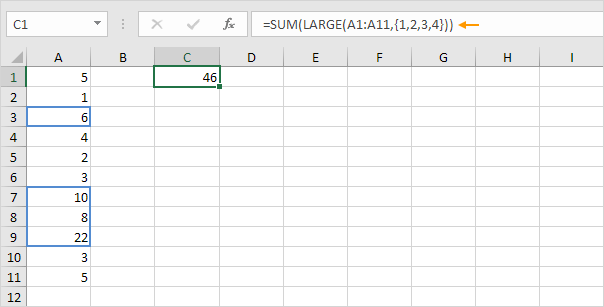3. A traditional TRANSPOSE function entered by preselecting the range E2:E4 and finished by pressing CTRL + SHIFT + ENTER.Explanation: the TRANSPOSE function converts a horizontal range to a vertical range, or vice versa.

4. The same function in Excel 365/2021, entered into a single cell, spills to neighboring cells.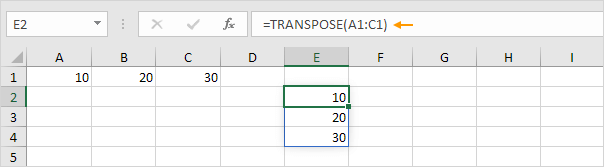### Spill Range

To refer to the values inside the blue rectangle, also called the spill range, always use the first cell and a hash character.

1. For example, the UNIQUE function below, entered into cell C1, extracts unique values.2. The COUNTA function below uses the spill range (C1#) to count unique values.3. If something is blocking the spill range, Excel displays the #SPILL! error.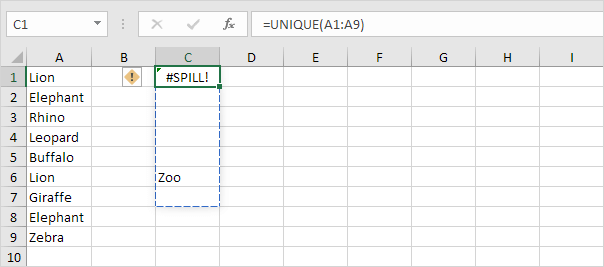Note: in this example, simply empty cell C6 to fix the #SPILL error.

Go to Next Chapter: Sort